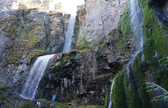# Labs

Electrostatics Kit > Products. 06.te.centripetal force. Physics 4A RMNiduaza: Lab 7: Centripetal Force. Purpose: To get familiar with electronic spreadsheets by using them in some simple applications Equipment: Computer with EXCEL software. Computer EXCEL Software Procedure: 1. 2. 3. F(x) = A sin(Bx + C) GIVEN CONSTANTS Cell Designation A = Amplitude = 5 J3 B = Frequency = 3 K3 C = Phase = π/3 (1.047) L3 x = Radians = from 0 to 10 in increments of 0.1 columns A, D & G For our Excel formula, we used the following to create the aforementioned function: (*Note: The dollar signs before and in between the cell location/s signifies an absolute cell reference, which is how we designated the constant values given in Excel) 4. 5. Upon running the analysis, our results are as follow: The analysis shows that the values for A, B and C are consistent with the original given values in part 3 of the procedure. 6.

For this scenario we chose to use the kinematic equation: r = position rf = ½ aΔt2 + V0Δt + ri where a = A, V0 = B, and ri = C A = acceleration of gravity = -9.8 m/s2 G4 B = Initial Velocity = 50 m/s H4 Results: My AP Physics Blog: Motion on an Incline Lab. Quantifying Motion: An Introduction to Kinematics Lab partners: Julia Bonner, Brandon Lee What is motion? Linear kinematics describes the movement of an object or group of objects without taking the causes of movement into consideration.

When we set out to study motion- how it works and what it tells us- it is important to understand a few fundamental mathematical concepts and formulas. We can measure motion through velocity, acceleration, and displacement, which differ from speed and time. Velocity, first of all, is defined as the rate of change, or the displacement over the change in time (vector quantity). Moving On: Motion on an Incline Setting up a controlled experiment involved using tools such as a Vernier Dynamics track which served as a ramp, a rolling cart, a motion detector, a data collection device, and LoggerPro software to measure how an object moves.

By completing this process, we wanted to determine how the velocity, acceleration were related. What Did We Learn? Clemson University Physics Labs. 141f11l02 [Physics Labs] Forces in Equilibrium Objective: To test the hypothesis that forces combine by the rules of vector addition, and to confirm that the net force acting on an object at rest is zero (Newton’s First Law). Equipment: Pasco Force table Four Fixed PulleysHooked Weight SetDual Range Force Sensor with Force Table BracketComputer with Signal Interface and PASCO Capstone Physical Principles: Sin, Cos, Tan Definitions of the Sine, Cosine, and Tangent of an Angle: Consider the acute (less than 90°) angle θ of the right triangle shown in Figure 1. The right (90°) angle is always formed by the adjacent and opposite sides. The size of the angle θ relates to the lengths of the right triangle’s three sides through the trigonometric functions Sine, Cosine, and Tangent.

Vector Addition Graphical method - Also known as the polygon method. Component method - Resolve the vector into components by choosing two perpendicular axes, X and Y, and projecting each vector onto these axes. Equilibrium Conditions so that A. B. McCulley's HTML 5 Physics Lab Simulations. Lab_6 [Physics Labs] Objectives To observe conservation of momentum in collision processes.To test kinetic energy conservation in elastic and inelastic collisions.To test momentum change as impulse.

Equipment Pasco dynamics track Dynamic track feet (as needed)Fixed pulley (to calibrate the Force Sensor)2 Carts - one with a spring and one without. They must be able to velcro together (they should not magnetically repel each other)3 Cart accessory blocks2 Motion SensorsForce Sensor and wooden block 1 cm thickElectronic Scale (to measure masses)Hooked weights (to calibrate the Force Sensor)Computer with Pasco 850 Signal Interface and PASCO Capstone software Principles It can be said that the impulse acting on an object is equal to the change in momentum of the object.

Where I is the vector impulse, the product of the force and the time that the force acts on the system. If the total kinetic energy KE of the setup is conserved, it is said that an elastic collision has occurred. Predictions Procedures Setup: Part 3. Centripetal Force Experiment: Lab Analysis. Centripetal Force By: Alexander Jones Abstract In this experiment Newton’s first and second laws of motion were used to study and verify the expression for the force, F, to be provided to mass, m, to execute circular motion.

Two experimental conditions were measured using 1) a simple pendulum and 2) a rotating table. To acquire data regarding force, period, and velocity of the experimental setups a force sensor and photogate motion sensor were employed. The data was then analyzed graphically and mathematical calculations were performed on the graphical data. Experimental Theory Newton’s laws are used to describe patterns of motion on masses. Applying a force parallel to the direction of motion will cause the velocity to either increase or decrease in magnitude, whereas a force applied to any angle will cause a change in magnitude and direction.

Figure I: Showing the similar triangles made by the velocities of equal magnitude compared to the triangle made by radii separated by angle Θ. Physlet Physics by Christian and Belloni: Illustration 3.4. Physlets run in a Java-enabled browser, except Chrome, on the latest Windows & Mac operating systems. If Physlets do not run, click here for help updating Java & setting Java security. Please wait for the animation to completely load. Consider the x direction. Notice that the x coordinate of the projectile (purple) is identical to the x coordinate of the blue object at every instant. What do you notice about the spacing between blue images? Now consider the y direction. Illustration authored by Aaron Titus with support by the National Science Foundation under Grant No. Projectile Motion.